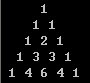# 从零开始学编程---第一步-C语言（二十五）1
1 1
1 2 1
1 3 3 1
1 4 6 4 1

for(i=0;i<5;i++)
{
a[i]=1;
a[i][i]=1;
}for(i=2;i<5;i++) 表示从第3行开始 ，因为1，2行没中间数
for(j=1;j<i;j++) 表示从第2个数开始，因为第一个数都是1，并且元素个数不能大于行数
a[i][j]=a[i-1][j-1]+a[i-1][j];

。。。

for(i=0;i<5;i++)
{
for(j=0;j<=i;j++)
printf("%d ",a[i][j]);

printf("\n");
}

#include <stdio.h>
main()
{
int a,i,j;

for(i=0;i<5;i++)   //存储两边的1
{
a[i]=1;
a[i][i]=1;
}

for(i=2;i<5;i++)   //存储中间的数子
for(j=1;j<i;j++)
a[i][j]=a[i-1][j-1]+a[i-1][j];

for(i=0;i<5;i++)   //打印
{
for(j=0;j<=i;j++)
printf("%d ",a[i][j]);

printf("\n");
}

}

#include <stdio.h>
main()
{
int a={0},i,j,k,n;

scanf("%d",&n);

for(i=0;i<n;i++)
a[i]=1;     //第一列全部赋值为1

for(i=1;i<n;i++)
for(j=1;j<=i;j++)
a[i][j]=a[i-1][j-1]+a[i-1][j];

for(i=0;i<n;i++)   //打印
{
for(k=n-1;k>i;k--)
printf(" ");
for(j=0;j<=i;j++)
printf("%d ",a[i][j]);

printf("\n");
}

}

for(i=0;i<n;i++)
a[i]=1;

for(i=1;i<n;i++)   这里就是从第2行开始了，不再是第3行
for(j=1;j<=i;j++)
a[i][j]=a[i-1][j-1]+a[i-1][j];

1
1 1
1 2 1
1 3 3 1
1 4 6 4 1

int a={0},这里给第一行第一个元素赋值0，赋值之后所有元素都为0，当然，你可以随便赋值一个数字，但是这里只能赋值一个元素，如果赋值两个元素的话，会产生结果错误，因为第2行的最后一个数字1是第一行第一个数加上后面那个数的结果，所以后面那个数必须为0

static int a;

#include <stdio.h>
main()
{
int a={1},i,j,k,n;

scanf("%d",&n);

for(i=1;i<n;i++)
{
a[i]=1;     //第一列全部赋值为1
for(j=1;j<=i;j++)
a[i][j]=a[i-1][j-1]+a[i-1][j];
}

for(i=0;i<n;i++)   //打印
{
for(k=n-1;k>i;k--)
printf(" ");
for(j=0;j<=i;j++)
printf("%d ",a[i][j]);

printf("\n");
}

}

for(i=1;i<n;i++)
{
a[i]=1;     //第一列全部赋值为1
for(j=1;j<=i;j++)
a[i][j]=a[i-1][j-1]+a[i-1][j];
}

int a={1}

int a;

int *p=a;

#include <stdio.h>
main()
{
"hello world";
}

int a,
&a就是取这个数组的地址，你猜的到，这个地址结果和a的结果一样，都是1000

a代表的是一个数组，在这里你不要管它就是地址1000，就当它是个名称吧
&a代表这个数组的一个整体的地址，虽然结果1000，但概念不一样

#include <stdio.h>
main()
{

char a={1,2,3,4,5};
printf("%d\n",a);
printf("%d\n",&a);
printf("%d\n",a+1);
printf("%d\n",&a+1);

}

1000
1000
1001
1005

a &a都是1000
a+1 是1001这个没错

&a并没有确切的地址，地址1000只是象征性，概念不一样，实际上数组的地址也只是象征性的存在，并非实实在在有，唉，好像越说越糊涂了

int a
a是这个数组的名称 做为一个数组的标志，它的1000是作为数组的入口
&a是这个数组的地址 它的1000是作为数组地址的存在（象征性的地址）

int a;

a,a,a
a,a,a

a是一个数组名，a数组包含2个元素，a,a

a,a是一维数组名，且数组名代表数组的首地址

a的地址怎么表示呢

int a

a 中有3个元素
a 中有3个元素

int a
*a代表什么？如果是一维数组，*a表示的首元素地址的值，也就是a的值

a和a都是a的地址，相当于a有两个别名

int a 这是普通的一维数组
a就是a的地址 a,a都有地址和空间（值）
int a 这是2维数组

a={a,a}

a等于a的地址，又等于a的值，a地址和值则都是&a（注意，只是这样看待，a实际没值也没地址）

*a 这是取值第一次 取a的值   而a就是首元素a的地址

*(*a)   由于*a的结果是地址，而不是值，所以还可以用*取值

*（*a）可以写为**a(这就是二级指针，指针的指针)

*a就是a,所以*a+1 就是a的地址

*(a+1)取a的值，结果还是地址，这里*(a+1)就等于a了

a+n就是第n行的数组名地址
*（a+n）就等价于数组名

*(*(a+3)+2)就是取a的值

*（a[i]+j）或*(*(a+i)+j)就是a[i][j]的值
*(a+i)和a[i]是等价的

*(a+i)+j和a[i]+j是等价的，表示a[i][j]的值
a表示a的地址，两者都是a的地址
*a表示a的值，这里*a等价于a,*a同样表示a的地址
a和*a的结果相同，但意义不相同，前者表示a的地址,后者表示a的值
a的地址和值都是a的地址（实际上a没有地址也没值）
a+i和*(a+i)的原理和a和*a一样
a+i表示a[i]的地址，而*(a+1)等价于a[i]

a=&a   *a=a
a+i=&a[i] *(a+i)=a[i]

&a[i]和a[i]结果一样

&a和a的结果一样

a+i=&a[i]=a[i]=*(a+1)=&a[i]

a只有两个元素，a,a

a也是2个元素，a,a

a相当于只有一个行数组，a相当于只有一个列数组（注意，行列数组还是有的，只不过只有一个元素而已）

#include <stdio.h>
main()
{
int a={{1,2,3},{4,5,6}};
int *p=a;
}

a只是行数组的数组名，也就是说a只包括a,a

int (*p)   这就是定义了一个数组指针

int a   这是个一维数组

int a   这是一个二维数组

a的行数组就是数组的数组，行数组里全部都是数组

a是一个数组的数组的数组名（就是一个数组里的元素都是数组）

a指向a这个数组，由于a也是数组里的一部分

int (*p)=a    就是数组指针p指向a，而a就是数组a的地址

p指向这个数组的第一个元素数组的地址，就有了数组名a的功能

int (*p)=a   就表示int (*p) 和a一样了

#include <stdio.h>
main()
{
int a={1,2,3,4,5};
int *p=&a;
printf("%d",*(p+1));
}

#include <stdio.h>
main()
{
int a={{1,2,3},{4,5,6}};
int *p=a;
printf("%d",*(p+1));
}

(5)
100%

(0)
0%